Different Transmission Techniques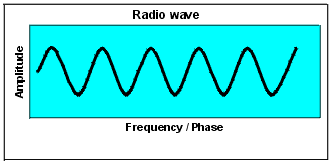This is the base diagram for a simple radio wave, compare AM & FM to this diagram on the left.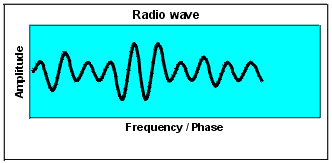AM stands for Amplitude Modulation, and is a type of transmission, that uses a carrier wave to carry your voice. Modulation is speech, Morse code, picture information, etc. When you talk through a radio microphone you are modulating. Refer to Diagram on left.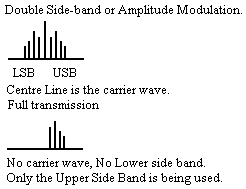SSB or single sideband is another type of transmission related to AM, only it uses one sideband and not a carrier wave like AM because AM is actually DSB, which is double sideband. You can either use USB, which is upper sideband or LSB, which is lower sideband. SSB sounds different on the radio compared to AM, especially over long distance communications.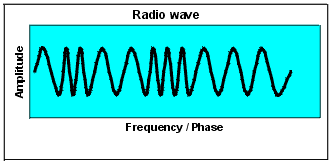FM stands for frequency modulation, and is another type of transmission, using a carrier but is slightly different. The frequency is modulated and the amplitude of the wave remains constant.

What the abbreviations VLF, LF, MF, HF, VHF, UHF, SHF, EHF stand for!
H = high, V = very, L = Low, U = ultra, F = frequency, S = super, E = extremely, and M = medium.

In the 'HF' radio band, being widely recognized to the general population as Short-wave band, the wavelengths are between 10 meters to 100 meters in length, so they are still highly frequent, hence the term High Frequency. At frequencies where we communicate using terrestrial microwave (satellites, and ground dish-to-dish) extremely short wavelength frequencies are used. This signifies Super High Frequency rate of wavelengths.

Digital Data
Digital data is in binary form, you have a 1, or a 0, and depending in which application it is applied 1 or 0 can mean ON or OFF. Generally 1 is ON or TRUE and 0 is OFF ir FALSE. Digital data is also encoded into suitable form for transmission throughout the radio spectrum.

Amplitude Modulation (AM) or Amplitude Shift Keying (ASK)
ASK transmits 1's and 0's by representing each one as a different amplitude at the same frequency. Therefore, the amplitude of a 1 is larger than the amplitude of the 0.

Frequency Modulation or Frequency Shift Keying
This time the amplitude does not change, but instead the frequency is altered. A 1 has a lower frequency than a 0. These frequencies are defined by the CCITT standard. The transmitter transmits a 0 at 1180Hz and a 1 at 980Hz. The receiver transmits a 0 at 1850Hz and a 1 at 1650Hz. (and the transmitter listens at these freqeinces)

Phase modulation or Phase Shift Keying (PSK) & Differential PSK (DPSK)
In this case, the frequency and amplitude are both kept constant. However, the phase of the sinewave is shifted to signify logic 0 and logic 1. Phase Coherent PSK uses two fixed signals, and a logic 0 is represented by a 180 degree phase shift and a logic 0 by a 0 degree phase shift. This is a complex method to demodulate for the receiver because the phase shifts are from an absolute value. Another method called Differential PSK exists (DPSK), which is a phase shift relative to the previous logic bit transmitted. Binary 0 is therefore a 90 degree phase change from the previous logic bit and binary 1 is a 270 degree phase change from the previous logic bit. In this case, the receiver only needs to detect the phase change which occured from the previous bit rather than off an absolute value that it has to know.

To achieve higher speed data communication a combination of PSK and AM can be used, which produces Quadrature Amplitude Modulation (QAM). This makes use of 0, 90, 180, 270 degree phase shifts together with ASK. These are used to produce bit rates of 2400, 4800 & 9600 bps.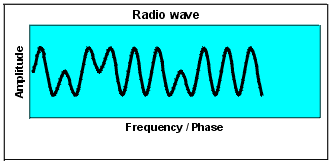PSK data signal

This Web page was last updated on Friday September 21, 2001

© 2001 Designed by Colin McCord